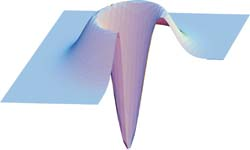Quantum information technology
A homodyne tomography tutorial

How can one determine a quantum state’s Wigner function? Although it cannot be measured directly as a probability density, all its marginal distributions can. Once we know all the marginal distributions associated with different quadratures – i.e. the Wigner function projections upon various vertical planes – we can reconstruct the Wigner function. This reconstruction procedure is very similar to that used by medical doctors in computer tomography – where one reconstructs a three-dimensional picture of a tissue anomaly from a set of two-dimensional X-ray images acquired from different angles.Fig. 1

So our goal is to determine the marginal distributions for all different “points of view”. Suppose we can measure the coordinate of our oscillator (for example, by taking its photograph at a given time). Repeating this procedure many times for a set of identical quantum states we obtain the marginal distribution pr(X) for quadrature X. This is, however, insufficient for tomographic reconstruction of the Wigner function: we need distributionsfor all values of q. In order to understand how to obtain these distributions we need to recall that our oscillator, well, oscillates. That is, our Wigner function rotates around the phase space origin with the oscillation frequency w. The marginal distribution pr(X) measured at the moment t = q / w is the same as the marginal distribution pr(Xq) measured at the moment t = 0. Therefore, instead of measuring the marginal distributions at a particular moment for all “view angles” we can measure marginal distribution associated with one view angle, but at different moments [Fig. 1].Fig. 2

Let us now remember that we work with light, not with mechanical oscillators. As we know from classical physics, these two physical systems are described by the same equations of motion. The role of the mechanical coordinate in the electromagnetic wave is played by the electric field. If we had an “electroscope” able to perform phase-sensitive measurements of the electric field in an electromagnetic mode, we would be able to reconstruct the “quantum portrait” of the mode’s quantum state – its Wigner function.

Unfortunately, such an “electroscope” does not exist. Typical oscillation frequencies of the light fields are hundreds of terahertz, and no one can build a detector that can follow such fast changes. Yet one can use a trick that allows to do phase-sensitive measurements of the electric field using regular, “slow” detectors. This trick is called balanced homodyne detection – here is its main idea.

Quantum objects are usually small, and electromagnetic fields that exhibit quantum properties are usually weak. Let us overlap the mode whose quantum properties we want to measure (the “signal” mode) with a relatively strong laser beam (the “local oscillator”) on a beam splitter [fig. 2]. If the electric fields in the two modes are Es and ELO, the fields emerging in the two beam splitter output ports are given byLet us now have both beam coming out of the beam splitter hit high-efficiency photodiodes. A photodiode is a device which generates electric current proportional to the intensity (not the amplitude) of the electromagnetic field incident on it. Our two photodiodes produce photocurrents that we subtract from each other. The photocurrent difference is given by;

since ELO >> Es, we neglect the quantum noise of the local oscillator field and find out that the photocurrent difference is proportional to the amplitude of the signal field – exactly the quantity we are looking for! By changing the relative optical phase of the local oscillator and signal waves, we measure the electric field at different phases. At each phase, we perform a multitude of electric field measurements (each time preparing an identical quantum state in the signal channel) thus obtaining the marginal distribution. A set of marginal distributions for various q’s will provide us with full information about the quantum state – and allows us to reconstruct its Wigner function and the density matrix.

An excellent introduction into nonclassical light and methods of its characterization is given in the textbook by U. Leonhardt “Measuring the quantum state of light”, CambridgeUniversity Press, 1997.

Our review paper on homodyne tomography

Gerd Breitenbach’s homodyne tomography page